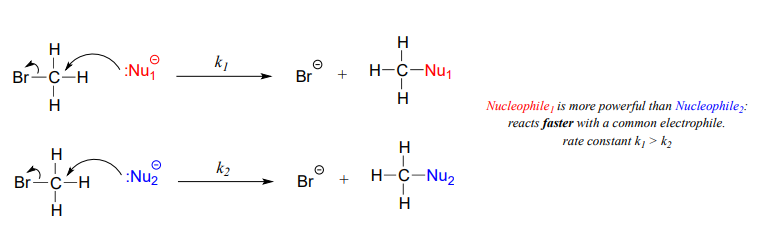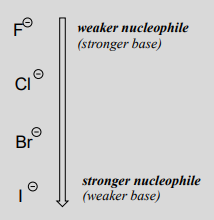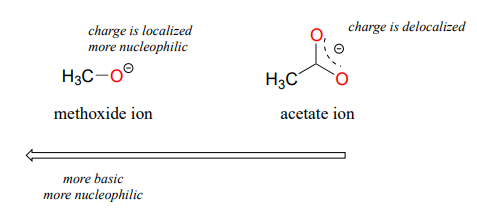# 3: Nucleophiles

•• Contributed by Tim Soderberg
• Emeritus Associate Professor of Chemistry at University of Minnesota Morris

## What is a nucleophile?

A nucleophile is an atom or functional group with a pair of electrons (usually a non-bonding, or lone pair) that can be shared. The same, however, can be said about a base: in fact, bases can act as nucleophiles, and nucleophiles can act as bases. What, then, is the difference between a base and a nucleophile?

A Brønsted-Lowry, as you will recall from chapter 7, uses a lone pair of electrons to form a new bond with an acidic proton. We spent much of chapter 7 discussing how to evaluate how basic a species is. Remember that when we evaluate basicity - the strength of a base - we speak in terms of thermodynamics: where does equilibrium lie in a reference acid-base reaction?We will spend much of this section discussing how to evaluate how nucleophilic a species is - in other words, its nucleophilicity. A nucleophile shares its lone pair of electrons with an electrophile - an electron-poor atom other than a hydrogen, usually a carbon. When we evaluate nucleophilicity, we are thinking in terms of kinetics - how fast does the nucleophile react with a reference electrophile?In both laboratory and biological organic chemistry, the most common nucleophilic atoms are oxygen, nitrogen, and sulfur, and the most common nucleophilic compounds and functional groups are water/hydroxide ion, alcohols, phenols, amines, thiols, and sometimes carboxylates.

In laboratory (non-biological) reactions, halide ($$I^-$$, $$Br^-$$, $$Cl^-$$, $$F^-$$) and azide ($$N_3-$$) anions are also commonly seen acting as nucleophiles in addition to the groups mentioned above.

Carbon atoms can also be nucleophiles - enolate ions (section 7.6) are common carbon nucleophiles in biochemical reactions, while the cyanide ion ($$CN^-$$) is just one example of a carbon nucleophile commonly used in the laboratory.Understanding carbon nucleophiles will be critical when we study, in chapters 12 and 13, the enzyme-catalyzed reactions in which new carbon-carbon bonds are formed in the synthesis of biomolecules such as DNA and fatty acids. In the present chapter, however, we will focus on heteroatom (non-carbon) nucleophiles.

Now, let's consider a number of factors that influence how nucleophilic an atom or functional group is. We'll start with protonation state.

## Protonation state

The protonation state of a group has a very large effect on its nucleophilicity. A negatively-charged hydroxide ion is much more nucleophilic (and basic) than a water molecule. In practical terms, this means that a hydroxide nucleophile will react in an $$S_N2$$ reaction with chloromethane several orders of magnitude faster than will a water nucleophile.

Likewise, a thiolate anion is more nucleophilic than a neutral thiol, and a neutral amine is nucleophilic, whereas an ammonium cation is not.

In a non-biological context, $$S_N2$$ reactions tend to occur with more powerful, anionic nucleophiles, where the nucleophile can be thought of as actively displacing ('pushing') the leaving group off the carbon. $$S_N1$$ reactions, in contrast, tend to be solvolysis reactions, with a weak, neutral nucleophile such as water or an alcohol.

Just as with basicity, there are predictable periodic trends associated with nucleophilicity. Moving horizontally across the second row of the periodic table, the trend in nucleophilicity parallels the trend in basicity:

##### The horizontal periodic trend in nucleophilicity

more nucleophilic $$NH_2$$- > $$OH$$- > $$F$$- less nucleophilic

more nucleophilic $$R$$-$$NH_2$$ > $$R$$-$$OH$$ less nucleophilic

Recall from section 7.3 that the basicity of atoms decreases as we move vertically down a column on the periodic table: thiolate ions are less basic than alkoxide ions, for example, and bromide ion is less basic than chloride ion, which in turn is less basic than fluoride ion. Recall also that this trend can be explained by considering the increasing size of the 'electron cloud' around the larger ions: the electron density inherent in the negative charge is spread around a larger volume, which tends to increase stability (and thus reduce basicity).

The vertical periodic trend for nucleophilicity is somewhat more complicated that that for basicity, and depends on the solvent in which the reaction is taking place. Take the general example of the $$S_N2$$ reaction below:where $$Nu^-$$ is one of the halide ions: fluoride, chloride, bromide, or iodide, and $$X$$ is a common leaving group. If this reaction is occurring in a protic solvent (that is, a solvent that has a hydrogen atom bonded to an oxygen or nitrogen - water, methanol and ethanol are protic solvents), then the reaction will go fastest when iodide is the nucleophile, and slowest when fluoride is the nucleophile, reflecting the relative strength of the nucleophile.

##### The vertical periodic trend in nucleophilicity in water and other protic solvents

(opposite of the trend in basicity!)This is the opposite of the vertical periodic trend in basicity (section 7.3), where iodide is the least basic. What is going on here? Shouldn't the stronger base, with its more reactive unbonded valence electrons, also be the stronger nucleophile?

As mentioned above, it all has to do with the solvent. Remember, we are talking now about the reaction running in a protic solvent like water. Protic solvent molecules form strong noncovalent interactions with the electron-rich nucleophile, essentially creating a 'solvent cage' of hydrogen bonds:

artwork neededFor the nucleophile to attack in an $$S_N2$$ reaction, the nucleophile-solvent hydrogen bonds must be disrupted - in other words, the nucleophilic electrons must 'escape through the bars' of the solvent cage. A weak base like iodide ion interacts weakly with the protons of the solvent, so these interactions are more readily disrupted. Furthermore, because the valence electrons on iodide ion are far from the nucleus, the electron cloud is polarizable - electron density can readily be pulled away from the nucleus, through the solvent cage and toward the electrophile.

A smaller, more basic anion such as fluoride is more highly shielded by stronger interactions with the solvent molecules. The electron cloud of the fluoride ion is smaller and much less polarizable than that of an iodide ion: in water solvent, the larger iodide ion is a more powerful nucleophile than the smaller fluoride ion.

##### Note

The above discussion of the vertical periodic trend in nucleophilicity applies to biochemical reactions, because the biological solvent is water. The picture changes for laboratory reactions if we switch to a polar aprotic solvent, such as acetone, which is polar enough to solvate the polar and ionic compounds in the reaction but is not a hydrogen bond donor, and does not form a strong 'solvent cage' like water does. In acetone and other polar aprotic solvents, the trend in nucleophilicity is the same as the trend in basicity: fluoride is the strongest base and the strongest nucleophile.

Structures of some of the most common polar aprotic solvents are shown below. These solvents are commonly used in laboratory nucleophilic substitution reactions.In biological chemistry, the most important implication of the vertical periodic trend in nucleophilicity is that thiols are more nucleophilic than alcohols. The thiol group in a cysteine amino acid residue, for example, is more nucleophilic than the alcohol group on a serine, and cysteine often acts as a nucleophile in enzymatic reactions. The thiol group on coenzyme A is another example of a nucleophile we will see often in enzymatic reactions later on. Of course, reactions with oxygen and nitrogen nucleophiles are widespread in biochemistry as well.## Resonance effects on nucleophilicity

Resonance effects also come into play when comparing the inherent nucleophilicity of different molecules. The reasoning involved is the same as that which we used to understand resonance effects on basicity (see section 7.3). If the electron lone pair on a heteroatom is delocalized by resonance, it is inherently less reactive - meaning less nucleophilic, and also less basic. An alkoxide ion, for example, is more nucleophilic and more basic than a carboxylate group, even though in both cases the nucleophilic atom is a negatively charged oxygen. In an alkoxide, the negative charge is localized on a single oxygen, while in the carboxylate the charge is delocalized over two oxygen atoms by resonance.The nitrogen atom on an amide is less nucleophilic than the nitrogen of an amine, due to the resonance stabilization of the nitrogen lone pair provided by the amide carbonyl group.##### Exercise 8.3.1

Which amino acid has the more nucleophilic side chain - serine or tyrosine? Explain.

## Steric effects on nucleophilicity

Steric hindrance is an important consideration when evaluating nucleophility. For example, tert-butanol is less potent as a nucleophile than methanol. The comparatively bulky methyl groups on the tertiary alcohol effectively block the route of attack by the nucleophilic oxygen, slowing the reaction down considerably (imagine trying to walk through a narrow doorway while carrying three large suitcases!).A final note: when it comes to comparing the rate of nucleophilic substitution reactions, the strength of the nucleophile only matters for $$S_N2$$ reactions. It is irrelevant for $$S_N1$$ reactions, because the rate-determining step (when the leaving group departs and a carbocation intermediate forms) does not involve the nucleophile.

Video tutorial: nucleophiles

##### Exercise 8.3.2

Which is the better nucleophile - a cysteine side chain or a methionine side chain? A serine or a threonine? Explain.

##### Exercise 8.3.3

In each of the following pairs of molecules/ions, which is expected to react more rapidly with CH3Cl in acetone solvent? Explain your choice.

1. phenolate (deprotonated phenol) or benzoate (deprotonated benzoic acid)?
2. water or hydronium ion?
3. trimethylamine or triethylamine?
4. chloride anion or iodide anion?
5. CH3NH- or CH3CH2NH2?
6. acetate or trichloroacetate?
7. aniline or 4-methoxyaniline?
8. phenolate or 2,6-dimethylphenolate?